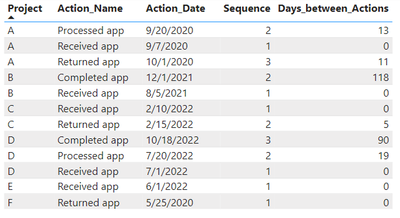cancel
Showing results for
Did you mean:Helper I

## Filter is Excluding Days Needed in Cumulative Total

Hi, all. I have this table with multiple projects.I used the formula below to calculate a new column called Fiscal Year, based on the following criteria:

1. Must be the MAX Sequence for the project
2. Must be an an Action_Name of either “Returned app” or “Completed app”
• If the first two criteria apply and the Action_Date is 10/01/2020 – 09/30/2021, then Fiscal Year = “FY21”
• If the first two criteria apply and the Action_Date is 10/01/2021 – 09/30/2022, then Fiscal Year = “FY22”
• If the first two criteria apply and the Action_Date is 10/01/2022 – 09/30/2023, then Fiscal Year = “FY23”
• If nothing applies, then BLANK

Fiscal Year =
VAR __Seq = [Sequence]
VAR __Project = [Project]
VAR __Action = [Action_Name]
VAR __Table =
FILTER ( 'Table', [Project] = __Project )
VAR __MaxSeq =
MAXX ( __Table, [Sequence] )
RETURN
IF (
__Seq = __MaxSeq
&& ( __Action = "Returned app"
|| __Action = "Completed app" ),
SWITCH (
TRUE (),
[Action_Date] >= DATE ( 2020, 10, 1 )
&& [Action_Date] <= DATE ( 2021, 9, 30 ), "FY21",
[Action_Date] >= DATE ( 2021, 10, 1 )
&& [Action_Date] <= DATE ( 2022, 9, 30 ), "FY22",
[Action_Date] >= DATE ( 2022, 10, 1 )
&& [Action_Date] <= DATE ( 2023, 9, 30 ), "FY23",
BLANK ()
),
BLANK ()
)

The problem with this formula is that it’s causing an error in the cumulative total of Days_between_Actions, since it excludes certain days from the calculation. The Days_between_Actions column is used to calculate the total number of days to complete a project (e.g., Project A took 24 days to complete [13 + 11 = 24]). The current formula for Fiscal Year calculates Project A as 11 days instead of the correct 24. How can I adjust the formula to ensure the Days_between_Actions column isn't affected?

1 ACCEPTED SOLUTIONCommunity Support

Hi  @PBInewbie17 ,

You can consider creating a measure to achieve cumulation:

``````Measure =
SUMX(
FILTER(ALLSELECTED('Table'),
'Table'[Action_Date]<=MAX('Table'[Action_Date])&&'Table'[Project]=MAX('Table'[Project])),[Days_between_Actions])``````

Result:Best Regards,

Liu Yang

If this post helps, then please consider Accept it as the solution to help the other members find it more quickly

2 REPLIES 2Community Support

Hi  @PBInewbie17 ,

You can consider creating a measure to achieve cumulation:

``````Measure =
SUMX(
FILTER(ALLSELECTED('Table'),
'Table'[Action_Date]<=MAX('Table'[Action_Date])&&'Table'[Project]=MAX('Table'[Project])),[Days_between_Actions])``````

Result:Best Regards,

Liu Yang

If this post helps, then please consider Accept it as the solution to help the other members find it more quicklyHelper I

There is still an issue. When I filter by Fiscal Year, the number of days in the Measure changes. It should remain the same. Please see the screenshot.